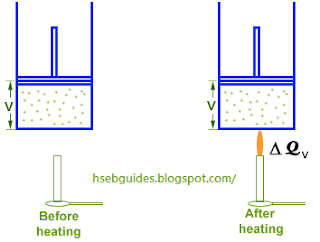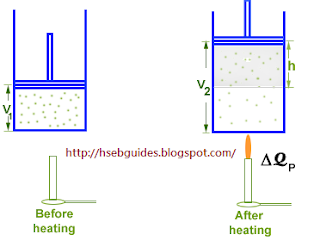# Molar Specific Heat At Constant Pressure

“The amount of heat energy required to raise the temperature of one mole of a gas by one Kelvin at constant pressure is called molar specific heat at constant pressure”.
It is denoted by Cp.

Mathematical expression
If DQp is the amount of heat is supplied at constant pressure to ‘n’ moles of a gas to increase the temperature by DT K, then
DQp = n CpDT
Or
Cp = D Qp /n D T
heat supplied at constant pressure is consumed in two purposes:

1. To raise the temperature of gas.
2. To do work against external pressure.

Show that: CP – CV = R
Consider ‘n’ moles of an ideal gas contained in a cylinder fitted with a frictionless piston. If the piston is fixed and the gas is heated, its volume remains constant and all the heat supplied goes to increase the internal energy of the molecules due to which the temperature of the gas increases. If DQv is the amount of heat supplied and DT is the rise in temperature then,
DQv = n CvD TThe pressure of the gas increases during this process, but no work is done because the volume is kept constant.
Hence D W = 0. applying first law of thermodynamics
Heat supplied = Increase in internal energy + Work done
DQv = D U + 0
DQv = D U
OR
nCvD T = D U

If the piston is free to move, the gas may be allowed to expand at a constant pressure. Let the amount of heat supplied is now is DQp. The addition of heat causes two changes in the system:• Increase in internal energy
• Work done against external pressure

According to the first law of thermodynamics:
DQ = DU + DW
{But DW = PDV} DQP = DU + PDV

Since DQp = nCpDT
and DU = nCvDT , therefore,
nCpDT = nCvDT + PDV………………..(1)
We know that PV = nRT
At T1 Kelvin: PV1 = nRT1 …..(a)
At T2 Kelvin: PV2 = nRT2…..(b)
Subtracting (a) from (b)
PV2 – PV1= nRT2 – nRT1 P(V2 – V1)= nR(T2 – T1) {(V2 – V1) = DV and (T2 – T1) = DT }
PDV = nRDT
Putting the value of PDV in equation (1)
nCpDT = nCvDT + nRDT nCpDT = nDT(Cv + R)Cp = (Cv + R)Cp – Cv = R